Function Repository Resource:

# CellularAutomatonFromNumber

Convert numbers specifying a cellular automaton into an explicit list of rules

Contributed by: Wolfram Physics Project Team
 ResourceFunction["CellularAutomatonFromNumber"][n] converts a cellular automaton specified with elementary rule number n into an equivalent list of rules. ResourceFunction["CellularAutomatonFromNumber"][n,k] converts a k-color cellular automaton with general nearest‐neighbor rule number n into an equivalent list of rules. ResourceFunction["CellularAutomatonFromNumber"][n,k,r] converts a cellular automaton specified with general rule number n,k colors and range r into an equivalent list of rules.

## Details and Options

The number of possible cellular automaton rules is as follows:
 elementary rules 256 1D general rules kk2r+1

## Examples

### Basic Examples (2)

Generate the list of rules corresponding to rule 30:

 In:=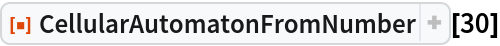Out=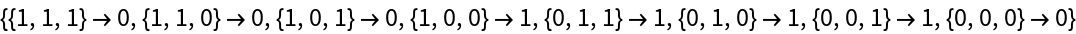Show that the two specifications are equivalent:

 In:=Out=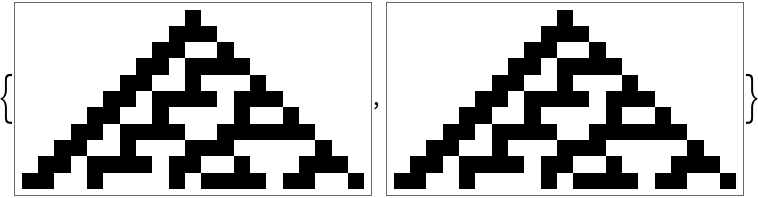### Scope (2)

Generate the rule representation of 3-color rule 679458:

 In:=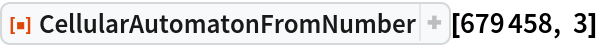Out=Generate the rule representation of a general range-r=2 rule:

 In:=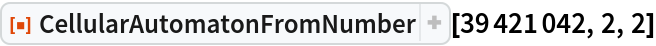Out=## Version History

• 1.0.0 – 12 August 2020